# Qualitative Analysis of State/Event Fault Trees Based on Interface Automata

Qualitative Analysis of State/Event Fault Trees Based on Interface Automata
School of Internet of Things, Nanjing University of Posts and Telecommunications, Nanjing 210003, China

College of Information Science and Technology, Nanjing Forestry University, Nanjing 210037, China

Corresponding Author Email:
hegaofeng@njupt.edu.cn
Page:
663-669
|
DOI:
https://doi.org/10.18280/ijsse.110606
22 May 2018
|
Accepted:
2 October 2019
|
Published:
28 December 2021
| Citation

OPEN ACCESS

Abstract:

State/Event Fault Tree (SEFT) can be used for safety modeling and assessment. However, SEFT does not provide adequate semantics for analyzing the minimal scenarios leading to system failures. In this paper, we propose a novel qualitative analysis method for SEFT based on interface automata. Firstly, we propose the concept of guarded interface automata by adding guards on interface automata transitions. Based on this model, we can describe the triggers and guards of SEFT simultaneously. Then, a weak bisimilarity operation is defined to alleviate the state space explosion problem. Based on the proposed guarded interface automata and the weak bisimilarity operation, the semantics of SEFT can be precisely determined. After that, a qualitative analysis process is presented on the basis of the formal semantics of SEFT, and the analyzing result is the minimal cut sequence set representing the causes of system failures. Finally, a fire protection system case study is illustrated step by step to demonstrate the effectiveness of our method.

Keywords:

state/event fault trees, minimal cut sequences, guarded interface automata, weak bisimilarity

1. Introduction

As safety critical systems are ubiquitous in our daily lives, failures of these systems will cause pollution of the environment, property losses, and even casualties . Thus, it is vital to assess the safety level and detect the key drawbacks of systems. To this end, safety analysis has been proposed. In order to perform safety analysis, fault tree analysis  is a widely accepted methodology, and there have been many methods developed for evaluating fault tree models. Generally, these methods can be categorized into quantitative and qualitative analysis [3, 4]. Specifically, qualitative analysis is used to identify the most critical components of the system. These critical components’ failures would cause the system to crash. To perform qualitative analysis, one needs to find out the Minimal Cut Sequences (MCSs) [5, 6], which form a minimal set of component events and states in a particular order that would cause the failure of a system. Based on MCSs, the most critical components can be identified efficiently.

In this paper, our main concern is the qualitative analysis for State/Event Fault Tree (SEFT) , which is a new kind of fault tree. Unlike the traditional fault tree , SEFT provides more flexible modeling capabilities for analyzing critical systems by distinguishing symbols of states and events . The occurrence of SEFT’s top event is related to the set of basic events and their sequences. Therefore, SEFT is more suitable for expressing safety critical scenarios of software-controlled systems.

As in fault tree analysis, one should first find out the minimal cut sequences (MCSs) to perform qualitative analysis for State/Event Fault Tree (SEFT). However, the SEFT’s component- and state-based natures make this task even harder. On the one hand, transitions cannot be triggered by states of a component, which can only allow or inhibit transitions. Consequently, we must model both triggers and guards when producing MCSs for SEFT. On the other hand, as lacking well-defined semantics, it is imperative to formally express the semantics of SEFT with the help of some formal models. And the chosen formal model should have component- and state-based natures. For example, Roth and Liggesmeyer  first translated SEFT to Extended Deterministic and Stochastic Petri Nets (eDSPNs) and captured the reachability graph based on eDSPNs. With the reachability graph, the qualitative analysis is then performed. However, this process requires two model translation steps (SEFT to eDSPNs and then to reachability graph) and a lot of manual interventions.

In this work, we present a novel solution for qualitatively analyzing SEFT. We first propose a new model called Guarded Interface Automata (GIA). Based on interface automata , GIA adds guards on transitions and can precisely describe the triggers and guards of transitions. Then, we define the semantics of SEFT’s logic gates with the help of guarded interface automata. Next, we provide a parallel composition and aggregation method to obtain the semantics of SEFT. Finally, we perform the qualitative analysis with the obtained semantics and illustrate the process by applying our method. Our approach only needs one step of model translation (SEFT to GIA). And the translated models can be almost automatically composited except that the order of composition should be determined manually. Therefore, less manual efforts are needed in our method, and the analyzing efficiency will be improved.

The remainder of the paper is organized as follows. We give an overview of related work of qualitative analysis for fault trees in Section 2. In Section 3, the weak bisimilarity operation of guarded interface automata is defined. In Section 4, we capture the semantics of SEFT and demonstrate the process of qualitative analysis. A case study is given step by step in Section 5. We discuss the proposed method in Section 6 and conclude and identify some future work in Section 7.

2. Related Work

We investigate the qualitative analysis method for State/Event Fault Tree in this work. Existing work on this topic is presented in two aspects: fault tree models and extraction of minimal cut sequences.

Models based on fault trees are widely accepted, which can show how influential factors contribute to some given hazard or accident . There are many types of fault trees supporting dynamic and component-based system modeling, among many others: Dynamic Fault Tree (DFT)  extends the basic fault tree with dynamic logic gates to describe dependencies of action sequences. The differences and categorizes of existing DFT variants are systematically uncovered in the study . Temporal Fault Tree (TFT)  extends the conventional fault tree with temporal logic, for example, the Interval Temporal Logic with continuous semantics and Duration Calculus . State/Event Fault Tree has been first presented by Kaiser . It provides a visual notation that combines elements from fault trees and state-based modeling techniques. Compared to the traditional fault trees, SEFT has the ability of visual distinction for states and events, which uses a graphical notation similar to Statecharts. Hence, the main advantage of SEFT is the usage of familiar symbols both from fault trees and Statecharts.

The minimum cut set analysis aims to find the failure causal chain of component systems. Tang and Dugan  first introduced minimal cut sequences for dynamic fault trees. However, they did not give detailed processes to generate minimal cut sequences. Liu et al.  provided an integrated method for all cut sequence generation in the dynamic fault tree. Xing et al. analyzed dynamic fault trees based on sequential binary decision diagrams . Assaf and Dugan diagnosed failed systems using the diagnostic decision tree (DDT) . In these works, the minimal cut sequences are constructed based on the dynamic fault tree.

Our purpose is to provide a new qualitative analysis method for SEFT. As described in Section 1, we have to consider both the component- and state-based natures of SEFT. Kaiser et al.  translated SEFT to eDSPNs (Extended Deterministic and Stochastic Petri Nets) to investigate the system failure probabilities. However, their work did not explain how to generate MCSs for SEFT. They only performed the quantitative analysis, not the qualitative analysis for SEFT. In fact, Kaiser  claimed that eDSPNs must be composed manually. This is because eDSPNs cannot possess the notion of interface. Therefore, it is challenging to perform qualitative analysis for SEFT based on eDSPNs.

Roth and Liggesmeyer  proposed a qualitative analysis method for SEFT by Kaiser . They first translated SEFT to eDSPNs according to Kaiser  and then captured the reachability graph based on eDSPNs. The qualitative analysis is performed based on the reachability graph lastly. During this process, they need two translation steps. In the first step, as eDSPNs do not have the notion of interface, it requires manual intervention to composite the elements’ formal semantic models. In the second step, the reachability graph is needed to be manually constructed from SEFT’s eDSPNs semantics model to mitigate state space explosion. This process requires two model translation steps and a significant amount of manual interventions.

In this paper, we design a new automatical and efficient method for qualitative analysis of SEFT. Our approach’s basic idea is to use interface automata to define SEFT elements’ precise semantics. Since interface automata automatically support models’ composition, we can obtain SEFT’s semantics by compositing its elements’ semantic model and finding out the MCSs without manual intervention.

3. Formal Description of SEFT’s Elements Based on Guarded Interface Automata

3.1 Informal description of SEFT model

In this section, we introduce the SEFT model informally. SEFT combines modeling elements from fault trees and statecharts. Differatiating from the fault tree, SEFT introduces distinct symbols for states and events, so SEFT is a state- and event-based model. In SEFT, logic gates can show how several causes relate to their common consequences. They can also visually build the complex trigger and guard structures, which are always connected by casual edges. In logic gates, states cannot trigger transitions, they can only inhibit or allow transitions. A state condition enabling a transition to occur is referred to as a guard, and the influenced transition can only happen as all guard conditions are evaluated to be true. Therefore, if there is more than one guard condition, they are ANDed. To reflect the state/event distinction, the input and output ports of gates are distinguished as state or event ports. The logic gates of SEFT are shown in Figure 1; there are five different kinds. The hollow box at the bottom of the logic gates represents the input port, and the solid box at the top represents the output port. The letter "S" in the box represents the state port, and the letter "E" represents the event port.

3.2 The guarded interface automata model

To model the semantics of SEFT, we proposed guarded interface automata by extending the interface automata . In this section, we formally describe the definition of guarded interface automata (GIA). Furthermore, we propose a weak bisimilarity operation to relieve the state space explosion problem during the GIA composition process.

3.2.1 Definition of GIA

Definition 1 (Guarded Interface Automaton). A GIA is a tuple P=(VP, $v_{P}^{\text {Init }}$, AP, IP, ΓP) where:

• VP is a finite set of states, where each state v$\in$VP.
• $v_{P}^{\text {Init }} \in$VP is the initial state.

## 1.png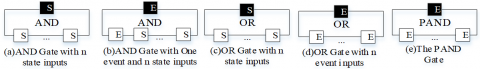Figure 1. Logic gates of SEFT

• AP is a set of all actions, partitioned into disjoint sets of input, output, and internal actions, which are denoted by $A_{P}^{I}$, $A_{P}^{O}$ and $A_{P}^{H}$, respectively.
• IP is a finite set of guards, each guard has the form of [φ], where φ is the formula defined as φ:=s|φ$\wedge$φ|φ$\vee$φ, s $\in$ {Guarded states of components in system}.
• ΓP $\in$ VP×AP×IP×VP is a set of transitions.

Figure 2 shows two guarded interface automata P and Q. In Figure 2(a), the GIA P indicates the assumption that the transition occurs when the message a is sent. The composable of GIA is described in Definition 2, and Definition 3 defines the product of GIA.

## 2.pngFigure 2. Examples of guarded interface automata

Definition 2 (Composable): Two guarded interface automata P and Q are composable if $A_{P}^{H} \bigcap A_{Q}=\varnothing$, $A_{P}^{I} \cap A_{Q}^{I}=\varnothing$, $A_{P}^{O} \bigcap A_{Q}^{O}=\varnothing$, $A_{Q}^{H} \bigcap A_{P}=\varnothing$.

Definition 3 (GIA Product): If P and Q are composable guarded interface automata, their product P// the GIA defined as:

• $V_{P \| Q}=V_{P} \times V_{Q}$.
• $V_{P \| Q}^{\text {init }}=\left(V_{P}^{\text {init }} \times V_{Q}^{\text {init }}\right)$.
• $A_{P \| Q}^{I}=\left(\mathrm{A}_{P}^{I} \cup \mathrm{A}_{Q}^{I}\right) \backslash$ shared $(\mathrm{P}, \mathrm{Q})$,

$A_{P \| Q}^{O}=\left(\mathrm{A}_{P}^{O} \cup \mathrm{A}_{Q}^{O}\right) \backslash$ shared $(\mathrm{P}, \mathrm{Q})$,

$A_{P \| Q}^{H}=\mathrm{A}_{P}^{H} \cup \mathrm{A}_{Q}^{H} \cup$ shared $(\mathrm{P}, \mathrm{Q})$.

• The set of guards is $I_{P \| Q}=I_{P} \bigcup I_{Q}$.
• $\Gamma_{P \| Q}=\left\{(\mathrm{s}, \mathrm{t}) \stackrel{a,[\mathrm{~g}]}{\longrightarrow}\left(\mathrm{s}^{\prime}, \mathrm{t}\right) \mid \mathrm{s} \stackrel{a,[\mathrm{~g}]}{\longrightarrow} s^{\prime} \wedge a \in A_{P} \backslash \mathrm{A}_{Q}\right\} \cup\{(\mathrm{s}, \mathrm{t})$

$\xrightarrow{a,[g]}(s,{t}')|t\xrightarrow{a,[g]}{t}'\wedge a\in {{A}_{Q}}\backslash {{A}_{P}}\}\bigcup$

$\left\{(\mathrm{s}, \mathrm{t}) \stackrel{a,[\mathrm{~g}]}{\longrightarrow}\left(\mathrm{s}^{\prime}, \mathrm{t}^{\prime}\right) \mid \mathrm{s} \stackrel{a,\left[\mathrm{~g}_{1}\right]}{\longrightarrow} s^{\prime} \wedge \mathrm{t}\right.$

$\stackrel{a,\left[\mathrm{~g}_{2}\right]}{\longrightarrow} t^{\prime} \wedge a \in$ shared $\left.(\mathrm{P}, \mathrm{Q})\right\}$.

3.2.2 Weak bisimilarity

Automata-based models usually suffer from combinatorial state explosion. To alleviate the state explosion problem, it is vital to find out weak bisimilarity relations of states. The weak bisimilarity of GIA is defined in Definition 4.

Definition 4 (Weak bisimilarity): Let P=(VP, $v_{P}^{\text {Init }}$, AP, IP, ΓP) be a guarded interface automaton. Let R be an equivalence relation on VP. Then R is a weak bisimilarity iff for all (s, t) $\in$ R, a$\in$AP, $s \stackrel{a,[g]}{\Rightarrow} s^{\prime}$ implies that there is a weak transition $t \stackrel{a,[g]}{\Rightarrow} t^{\prime}$ with (s', t') $\in$R.

Two guarded interface automata P and Q are weakly bisimilar, written P≈Q, if they are contained in some weak bisimilarity B. Weak bisimilarity for a GIA is defined as the union of all weak bisimilaritys on P:

• Ρ=$\cup${R|R is a weak bisimilarity on P}.

Our notion of weak bisimilarity for guarded interface automata is similar to the one for the interactive process . As pointed out in literature , weak bisimilarity is substitutive. Substitutivity ensures that equivalent components can be exchanged by each other without affecting the behavior of the composition process. Notably, our notion of weak bisimilarity enjoys the expected properties: weak bisimilarity is a congruence with respect to parallel composition.

Let P1 and P2 be two GIA with identical actions and guards, let P3 be a GIA composable with P1 and P2. We have:

1) P1P2 implies P1//P3P2//P3,

2) P1P2 implies P3//P1P3//P2.

As an example, the parallel composition and aggregation result of GIA P and Q in Figure 2 is shown in Figure 3.

## 3.png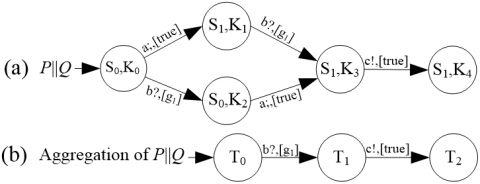Figure 3. Parallel composition and aggregation result of Figure 2

Furthermore, we use sequential failure symbol “→” to express the cut sequence of components’ events. From the behavior $v_{0} \stackrel{a_{0},\left[g_{0}\right]}{\longrightarrow} v_{1} \stackrel{a_{1},\left[g_{1}\right]}{\longrightarrow} \ldots \stackrel{a_{n-1},\left[g_{n-1}\right]}{\longrightarrow} v_{n}$, we can capture the cut sequence as: a0, [g0]→a1, [g1]→an-1, [gn-1].

3.3 Semantics for SEFT’s elements

This subsection provides the GIA semantics for each SEFT logic gate. The input of event type in a logic gate can be considered as the trigger event, and the input of state type in a logic gate can be considered as the guard. $\left[\!\left[ \left. ... \right]\!\right] \right._{E L T}$ is a function representing the semantics of logic gates. We show the GIA of the logic gates as follows.

• GIA model of state-AND Gate. Figure 4(a) expresses the semantics of the n input state-AND Gate, i.e., S1=[S2$\wedge$...$\wedge$Sn].
• GIA model of event/state-AND Gate. Figure 4(b) expresses the semantics of the one event input and n state input AND Gate (including state-AND Gate and event/state-AND Gate), i.e. function $\left[\!\left[ \left. {(event / state)-AND} \right]\!\right] \right._{E L T}: A^{n} \rightarrow G I A$ takes the output, one event input and n state inputs of the logic gate as parameters. The event/state-AND Gate fires if event input is triggered and state guards are satisfied.
• GIA model of state-OR Gate. Figure 4(c) expresses the semantics of the n input state-OR Gate, i.e., $S_{1}=\left[S_{2} \vee \ldots \vee S_{n}\right]$.
• GIA model of event-OR Gate. Figure 4(d) expresses the semantics of the n event inputs OR Gate, i.e. function $\left[\!\left[ \left. {(OR, n)} \right]\!\right] \right._{E L T}: A^{n} \rightarrow G I A$, takes the output and n event inputs of the logic gate as parameters.
• GIA model of PAND Gate. Figure 4(e) expresses the semantics of the n inputs PAND Gate, i.e. function $\left[\!\left[ \left. {(P A N D, n)} \right]\!\right] \right._{E L T}: A^{n} \rightarrow G I A$, takes the output and n event inputs of the logic gate as parameters.

## 4.png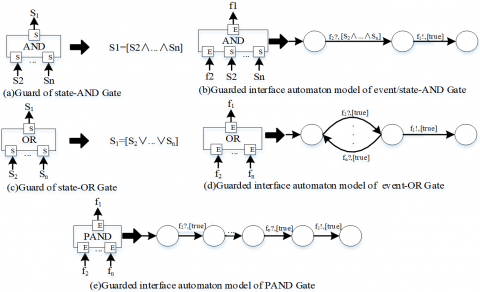Figure 4. GIA model for logic gates. The left side of the broad arrow indicates the logic gate of SEFT, and the right side represents the formal representation of the logic gate. Note that the formalized description of state-AND Gate and state-OR Gate is a guard, and the result of the formal representation of event/state-AND Gate, event-OR Gate, and PAND Gate is a guarded interface automaton

We have defined the individual GIA models for each SEFT element. We now can convert any given SEFT into the corresponding GIA. The method for generating SEFT’s semantics and minimal cut sequence will be presented in Section 4.

4. Minimal Cut Sequence Generation

The technique of compositional aggregation consists of composing a larger model out of smaller ones and aggregating submodels after each compositional step. The compositional aggregation methodology can combine a GIA set into a single GIA. Hence, we can transform SEFT’s logic gates into a GIA set and obtain the final semantics of a SEFT model.

4.1 Weak bisimilarity classes partition approach

When defining the semantics of SEFT’s logic gates, the output of a logic gate is formally described by the output action of GIA. Since these output actions added to derive semantics of SEFT are not the events of components, they do not influence the system behavior. Namely, they become internal actions and do not affect the qualitative analysis result. Consequently, these internal actions should be removed to reduce the state space of the compositional result.

Weak bisimilarity is substitutive with parallel composition, which lays the foundation for state space reduction. Substitutivity ensures that equivalent components can be exchanged by each other. And the exchange does not affect the behavior of the composite process. Thus, the weak bisimilarity technique can be used to reduce specific internal actions after the composition is completed. The state space reduction process of GIA can be divided into two phases: weak bisimilarity classes partition and state space aggregation, where the former one is the crucial part and the state space aggregation is easy to implement. Therefore, in the following, we mainly introduce the weak bisimilarity classes partition method in detail.

Algorithm 1 shows the weak bisimilarity classes partition process. The basic idea of Algorithm 1 is described as follows. First of all, the state space of GIA is partitioned into two parts according to whether the states are stable or not. In this paper, a state with no outgoing internal or output transitions is considered stable. Then every equivalence class is further partitioned by the left actions and guards of GIA. The algorithm ends when all the actions have been used. Finally, the state space of GIA is partitioned into several equivalence classes.

 Algorithm 1. Compute weak bisimilarity classes $\gamma_{o}(P,(a,[g]), C):= \begin{cases}\text { true } \text { if thereis } \quad P^{\prime} \in C \quad \text { suchthat } \quad P \stackrel{a,[g]}{\Rightarrow} P^{\prime}. \\ \text { false } \quad \text { otherwise. }\end{cases}$ IRefine $(P a r t,(a,[g]), C):$ $=\left(\cup_{X \in P a r t}\left(\cup_{v \in\{\text {true, false\} }}\left\{\left\{P \in X \mid \gamma_{O}(P,(a,[g]), C)=v\right\}\right\}\right)\right)-\{\phi\}$ Input: Guarded interface automaton $P=\left(V_{P}, v_{P}^{\text {Init }}, A_{P}, I_{P}, \Gamma_{P}\right)$ Output: VP/≈. Function: Compute weak bisimilarity classes of GIA P. Substitute specific internal action of GIA with τ. Part:={VP}; $\operatorname{Spl}:=\left(A_{P},\left[I_{P}\right]\right) \times\left\{V_{P}\right\}$; repeat Choose ((a,[g]),C) in Spl; Old:=Part; Part:=Irefine (Part,(a,[g]),C); New:=Part-Old; Spl:= $(\operatorname{Spl}-\{(a,[g]), C\}) \cup\left(\left(A_{P},\left[I_{P}\right]\right) \times N e w\right)$; Until Spl is empty Return Part.

## 5.png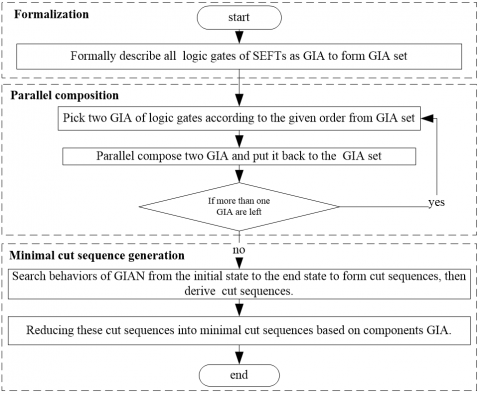Figure 5. MCS generation process of SEFT

The body of Algorithm 1 consists of two parts. To begin with, the initial operation is performed. The states of GIA are partitioned into stable states and non-stable states. Also, Spl is modified, i.e., Spl:=Act×{S}. After that, the main loop of the algorithm executes. The function of this loop is selecting one action from Spl and partitioning the existing equivalence classes. This loop ends when Spl is empty. The time complexity of this algorithm is O(n3), where n is the number of states in the GIA.

When the state space partitioning is completed, aggregation operation can be used to reduce state space of GIA: it processes every equivalence class respectively by merging the states to form a new state instead. In this way, we obtain a GIA model, the state space of which is smaller than before, and the behavior is weak bisimilar to the initial GIA model. For example, the aggregation result of Figure 3(a) is depicted in Figure 3 (b).

After the composition and aggregation of all guarded interface automata of SEFT logic gates, we can obtain a guarded interface automaton that represents the semantics of SEFT, from which we can analyze the MCSs.

4.2 SEFT cut sequences generation process

To analyze cut sequences, we make the initial state of the guarded interface automaton that represents the semantics of SEFT as the source state and the top event of SEFT destination states as the end state. Further, we use the existing depth-first searching algorithm to search all the behaviors from the source state to the end state. After that, we induce cut sequences by combining all the action sequences and the state guards on transitions of every behavior. Finally, the MCS set of SEFT can be achieved by reducing the cut sequences to several minimal cut sequences. The process flow of the method we proposed is shown in Figure 5.

5. Case Study

A SEFT model of a fire protection system is shown in Figure 6 . The hazard described by this SEFT is that when the smoke detector and the heat detector detect fire successively, and the water deluge system fails, then a fire might break out. The top event represents the failure of the entire system. The output of logic gate OR2 represents water deluge system failure, and the output of the logic gate PAND gate represents that fire detected.

## 6.png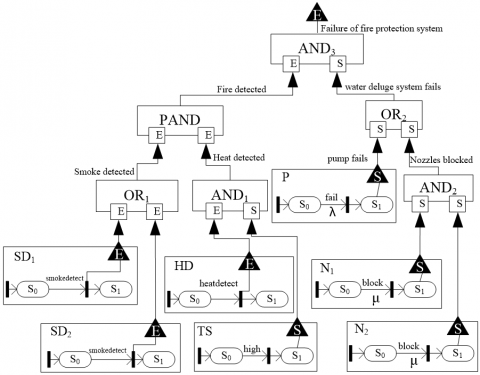Figure 6. The SEFT model of a fire protection system

## 7.pngFigure 7. Guarded interface automata of logic gates

## 8.png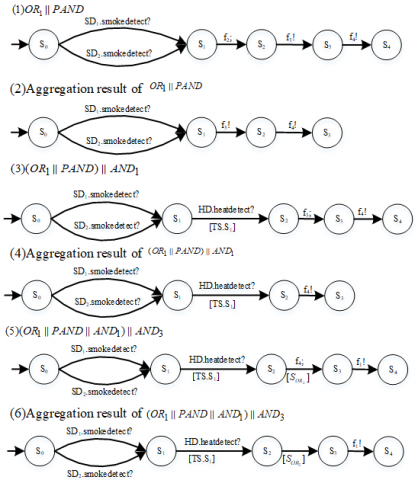Figure 8. Parallel composition and aggregation process

We first described logic gates semantics based on GIA, and the results are shown in Figure 7. After that, the complete semantics of the SEFT can be generated by composing all the guarded interface automata expressing the semantics of logic gates in Figure 7. In this case, the order adopted for GIA composition is OR1//PAND//AND1//AND3. Figure 8 shows the composition and aggregation process.

As shown in Figure 8, in every step, two guarded interface automata are selected to be composited. The result guarded interface automaton is further processed to reduce internal action by using the weak bisimilarity operation. After that, it is composited with other guarded interface automata in the next steps. The composition process ends when there is only one GIA left.

Finally, we obtain a result GIA as Figure 8(6). From this GIA, it is easy to get cut sequences shown as follows:

1) $\left(\mathrm{SD}_{1} . \mathrm{smokedetect} \rightarrow H D . h e a t d e t e c t ?\right) \wedge\left[T S . S_{1}\right] \wedge\left[S_{O R_{2}}\right]$

2) $\left(\mathrm{SD}_{2} . \mathrm{smokedetect} \rightarrow H \mathrm{D} . \mathrm{smokedetect} ?\right) \wedge\left[T S . S_{1}\right] \wedge\left[S_{O R_{2}}\right]$.

By substituting all guards in cut sequences by state guard expressions, we can obtain the minimal cut sequences by reducing all Ú symbols. The obtained minimal cut sequences are listed as the following:

1) $\left(\mathrm{SD}_{1} . \mathrm{smokedetect} \rightarrow H \mathrm{D} .\right.$ heatdetect?) $\wedge\left[T S . S_{1} \wedge P . S_{1}\right]$

2) $\left(\mathrm{SD}_{1} .\right.$ smokedetect $\rightarrow H$D.heatdetect?) $\wedge\left[T S . S_{1} \wedge N_{1} . S_{1} \wedge N_{2} . S_{1}\right]$

3) $\left(\mathrm{SD}_{2} . \mathrm{smokedetect} \rightarrow H\right.$D. heatdetect? $) \wedge\left[T S . S_{1} \wedge P . S_{1}\right]$

4) $\left(\mathrm{SD}_{2}\right.$.smokedetect $\rightarrow H \mathrm{D}$.smokedetect?) $\wedge\left[T S . S_{1} \wedge N_{1} . S_{1} \wedge N_{2} . S_{1}\right]$.

Based on these minimal cut sequences, we can obtain all minimal scenarios causing system failures. It is obvious that HD.smokedetect and TS.S1 are the key events and states because they exist in every minimal cut sequence.

6. Discussion

The advantage of our method is that it only needs one step of model translation, and the translated models can be automatically composited. Hence, the analyzing efficiency will be significantly improved. For comparison, we use the SEFTAnalyzer proposed by Roth and Liggesmeyer  to analyze the case system depicted in Figure 6. The experimental results are listed in Table 1. Compared to SEFTAnalyzer, the analysis results of our method are the same. Namely, both methods can find out the cut sequences of SEFT. For translation steps and manual operations, our approach only needs one step translation and only needs human interventions in the translation from SEFT to GIA (i.e., determining the composition orders manually). While SEFTAnalyzer is more complicated because it needs two steps of translation and several human interventions in different phases. For example, human interventions must be included in the translation from SEFT and eDSPNs, and in the generation of reachability graph to form complete SEFT’s semantics in SEFTAnalyzer. Therefore, the process steps of our method are more straightforward, and our method is quicker than SEFTAnalyzer (5 min vs. 15 min). The analyzing time is obtained by performing a complete analysis for the system depicted in Figure 6 with our method and SEFTAnalyzer.

The limitation of the proposed method is that the composition orders must be determined manually. This may be addressed by matching the models’ inputs and outputs iteratively. If a model’s output is accurately the input of another model, these two models can be composited and become an internal action. By repeating this, the composition orders may be generated automatically. We left it as our future work.

Table 1. Comparison of our method with SEFTAnalyzer 

 Method Translation steps Manual operations Analyzing results Analyzing time SEFTAnalyzer Two steps: from SEFT to eDSPNs, and from eDSPNs to Reachability graph composing elements’ eDSPNs models to form the complete SEFT’s semantics the same as our method 15 min Our method Only one step: from SEFT to GIA determining the composition orders 4 minimal cut sequences 5 min
7. Conclusions

In this paper, we presented a method for qualitatively analyzing SEFT. We propose the guarded interface automata to give precise semantics for SEFT’s logic gates. The semantics of SEFT is given by composing all the logic gates. During this process, we reduce the state-space of the compositional result by using aggregation methodology. We also present a cut sequences generation method. The obtained minimal cut sequences represent the shortest paths that trigger the critical event of the corresponding SEFT. As for future work, we are looking for more benchmark example State/Event Fault Trees that can be used to further test and improve our method.

Acknowledgment

This work is supported by National Natural Science Foundation of China under grants 61802192 and 61702282, Fundamental Research Funds for the Central Universities, NUAA, under the grant NJ2020022.

References

 Laplante, P.A., DeFranco, J.F. (2017). Software engineering of safety-critical systems: Themes from practitioners. IEEE Transactions on Reliability, 66(3): 825-836. https://doi.org/10.1109/TR.2017.2731953

 Ruijters, E., Stoelinga, M. (2015). Fault tree analysis: A survey of the state-of-the-art in modeling, analysis and tools. Computer Science Review, 15: 29-62. https://doi.org/10.1016/j.cosrev.2015.03.001

 Hiraoka, Y., Murakami, T., Yamamoto, K., Furukawa, Y., Sawada, H. (2016). Method of computer-aided fault tree analysis for high-reliable and safety design. IEEE Transactions on Reliability, 65(2): 687-703. https://doi.org/10.1109/TR.2015.2513050

 Kabir, S. (2017). An overview of fault tree analysis and its application in model based dependability analysis. Expert Systems with Applications, 77: 114-135. https://doi.org/10.1016/j.eswa.2017.01.058

 Tang, Z., Dugan, J.B. (2004). Minimal cut set/sequence generation for dynamic fault trees. In Annual Symposium Reliability and Maintainability, 2004-RAMS, Los Angeles, CA, USA, pp. 207-213. https://doi.org/10.1109/RAMS.2004.1285449

 Chaux, P.Y., Roussel, J.M., Lesage, J.J., Deleuze, G., Bouissou, M. (2013). Towards a unified definition of minimal cut sequences. IFAC Proceedings Volumes, 46(22): 1-6. https://doi.org/10.3182/20130904-3-UK-4041.00013

 Kaiser, B., Gramlich, C., Förster, M. (2007). State/event fault trees—A safety analysis model for software-controlled systems. Reliability Engineering & System Safety, 92(11): 1521-1537. https://doi.org/10.1016/j.ress.2006.10.010

 Roth, M., Liggesmeyer, P. (2013). Qualitative analysis of state/event fault trees for supporting the certification process of software-intensive systems. In 2013 IEEE International Symposium on Software Reliability Engineering Workshops (ISSREW), Pasadena, CA, USA, pp. 353-358. https://doi.org/10.1109/ISSREW.2013.6688920

 De Alfaro, L., Henzinger, T.A. (2001). Interface automata. ACM SIGSOFT Software Engineering Notes, 26(5): 109-120. https://doi.org/10.1145/503271.503226

 Čepin, M., Mavko, B. (2002). A dynamic fault tree. Reliability Engineering & System Safety, 75(1): 83-91. https://doi.org/10.1016/S0951-8320(01)00121-1

 Junges, S., Guck, D., Katoen, J.P., Stoelinga, M. (2016). Uncovering dynamic fault trees. In 2016 46th Annual IEEE/IFIP International Conference on Dependable Systems and Networks (DSN), Toulouse, France, pp. 299-310. https://doi.org/10.1109/DSN.2016.35

 Schellhorn, G., Thums, A., Reif, W. (2002). Formal fault tree semantics. In Proceedings of The Sixth World Conference on Integrated Design & Process Technology, Pasadena, CA, pp. 1-8.

 Palshikar, G.K. (2002). Temporal fault trees. Information and Software Technology, 44(3): 137-150. https://doi.org/10.1016/S0950-5849(01)00223-3

 Kaiser, B. (2006). State event fault trees: A safety and reliability analysis technique for software controlled systems. Ph.D. dissertation, University Kaiserslautern.

 Liu, D., Xing, W., Zhang, C., Li, R., Li, H. (2007). Cut sequence set generation for fault tree analysis. In International Conference on Embedded Software and Systems, pp. 592-603. https://doi.org/10.1007/978-3-540-72685-2_55

 Xing, L., Shrestha, A., Dai, Y. (2011). Exact combinatorial reliability analysis of dynamic systems with sequence-dependent failures. Reliability Engineering & System Safety, 96(10): 1375-1385. https://doi.org/10.1016/j.ress.2011.05.007

 Assaf, T., Dugan, J.B. (2004). Diagnostic expert systems from dynamic fault trees. In Annual Symposium Reliability and Maintainability, 2004-RAMS, Los Angeles, CA, USA, pp. 444-450. https://doi.org/10.1109/RAMS.2004.1285489

 Xu, B., Huang, Z., Hu, J., Wei, O., Zhou, Y. (2013). Minimal cut sequence generation for state/event fault trees. In Proceedings of the 2013 Middleware Doctoral Symposium, pp. 1-6. https://doi.org/10.1145/2541534.2541592

 Hermanns, H. (2002). Interactive Markov chains: And the quest for quantified quality. Berlin, Heidelberg: Springer-Verlag.

 Andrews, J.D. (2013). Tutorial. www.fault-tree.net, November 2013.